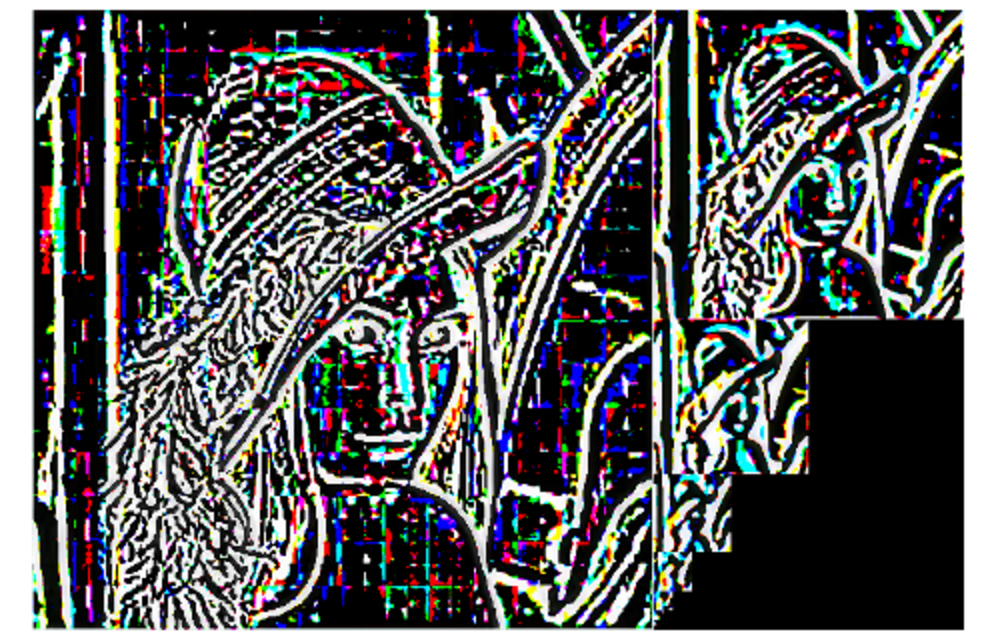# Scale Space v.s. Image Resolution

Posted by GwanSiu on August 24, 2017

## 1. 什么是尺度空间？

from skimage import data, filters,io
import matplotlib.pyplot as plt
%matplotlib inline

img1 = filters.gaussian(image, sigma=1.0)
img2 = filters.gaussian(image, sigma=2.0)
img3 = filters.gaussian(image, sigma=3.0)

plt.figure('gaussian',figsize=(8,8))
plt.subplot(221)
plt.imshow(image)
plt.axis('off')
plt.title('original image')
plt.subplot(222)
plt.imshow(img1)
plt.axis('off')
plt.title('gaussian kernel with sigmma=1.0')
plt.subplot(223)
plt.imshow(img2)
plt.axis('off')
plt.title('gaussian kernel with sigmma=2.0')
plt.subplot(224)
plt.imshow(img3)
plt.title('gaussian kernel with sigmma=3.0')
plt.axis('off')## 4. 图像金字塔

import matplotlib.pyplot as plt

from skimage import data, transform,io
from skimage.transform import pyramid_gaussian
import numpy as np

image = transform.resize(image, [512,512])
rows, cols, dim = image.shape
print(rows,cols)
pyramid = tuple(pyramid_gaussian(image, downscale=2,sigma=3))

composite_image = np.zeros((rows, cols + cols // 2, 3), dtype=np.double)

composite_image[:rows, :cols, :] = pyramid

i_row = 0
for p in pyramid[1:]:
n_rows, n_cols = p.shape[:2]
composite_image[i_row:i_row + n_rows, cols:cols + n_cols] = p
i_row += n_rows

fig, ax = plt.subplots()
ax.imshow(composite_image)
plt.axis('off')
plt.show()import matplotlib.pyplot as plt

from skimage import data, transform,io
from skimage.transform import pyramid_gaussian, pyramid_laplacian
import numpy as np

image = transform.resize(image, [512,512])
rows, cols, dim = image.shape
print(rows,cols)
pyramid = tuple(pyramid_laplacian(image, downscale=2,sigma=3))

composite_image = np.zeros((rows, cols + cols // 2, 3), dtype=np.double)

composite_image[:rows, :cols, :] = pyramid

i_row = 0
for p in pyramid[1:]:
n_rows, n_cols = p.shape[:2]
composite_image[i_row:i_row + n_rows, cols:cols + n_cols] = p
i_row += n_rows

fig, ax = plt.subplots()
ax.imshow(composite_image)
plt.axis('off')
plt.show()